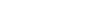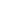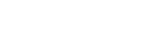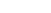Get inspired by the success stories of our students in IIT JAM MS, ISI  MStat, CMI MSc DS.  Learn More

# Symmetry, Counting, and Partition | ISI MStat PSB 2015 Problem 4This problem is an application of the non negative integer solution and the symmetry argument. This is from ISI MStat 2015 PSB Problem 4.

## Problem

Suppose 15 identical balls are placed in 3 boxes labeled A, B and C. What is the number of ways in which Box A can have more balls than
Box C?

### Prerequisites

• Symmetry Argument
• Number of non negative integer solutions tois. Not sure why? Search it up!

## Solution ( No Algebra )

There are three possible cases.

• Box A has more balls than Box C.
• Box C has more balls than Box A.
• Box A has the same number of balls as Box C.

The symmetry argument

The number of ways in which Box A has more balls than Box C = The number of ways in which Box C has more balls than Box A.

Isn't that very obvious, since the balls are not biased towards boxes. Why will they be?

Total Number of Ways = The number of ways in which Box A has more balls than Box C + The number of ways in which Box C has more balls than Box A + The number of ways in which Box A has the same number of balls as Box C.

The number of ways in which Box A has the same number of balls as Box C = 8 right?

Why? They can have either 0 ball each, 1 ball each, 2 balls each, ..., 7 balls each at most.

Therefore, The number of ways in which Box A has more balls than Box C =. []

### Challenge Problem

Suppose 15 identical balls are placed in 3 boxes labeled A, B, and C.
What is the number of ways in which Box A have no fewer balls than
Box B and Box B have no fewer balls than Box C?

This is related to the topic of mathematics called Partitions of Numbers.

This problem is an application of the non negative integer solution and the symmetry argument. This is from ISI MStat 2015 PSB Problem 4.

## Problem

Suppose 15 identical balls are placed in 3 boxes labeled A, B and C. What is the number of ways in which Box A can have more balls than
Box C?

### Prerequisites

• Symmetry Argument
• Number of non negative integer solutions tois. Not sure why? Search it up!

## Solution ( No Algebra )

There are three possible cases.

• Box A has more balls than Box C.
• Box C has more balls than Box A.
• Box A has the same number of balls as Box C.

The symmetry argument

The number of ways in which Box A has more balls than Box C = The number of ways in which Box C has more balls than Box A.

Isn't that very obvious, since the balls are not biased towards boxes. Why will they be?

Total Number of Ways = The number of ways in which Box A has more balls than Box C + The number of ways in which Box C has more balls than Box A + The number of ways in which Box A has the same number of balls as Box C.

The number of ways in which Box A has the same number of balls as Box C = 8 right?

Why? They can have either 0 ball each, 1 ball each, 2 balls each, ..., 7 balls each at most.

Therefore, The number of ways in which Box A has more balls than Box C =. []

### Challenge Problem

Suppose 15 identical balls are placed in 3 boxes labeled A, B, and C.
What is the number of ways in which Box A have no fewer balls than
Box B and Box B have no fewer balls than Box C?

This is related to the topic of mathematics called Partitions of Numbers.

This site uses Akismet to reduce spam. Learn how your comment data is processed.

### Knowledge Partner